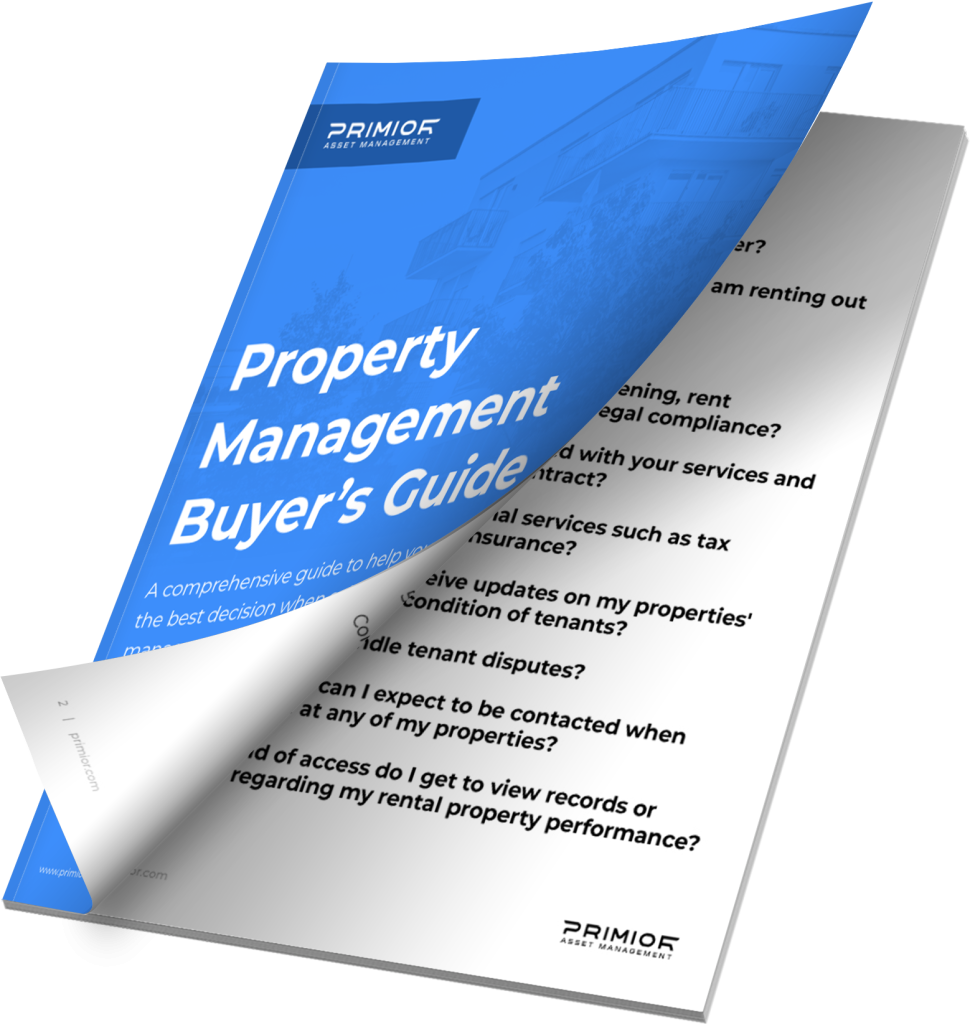/
Compound Interest Calculator
4.9
4.9/5

# Compound Interest Calculator

## Noted by Warren Buffet as the eighth wonder of the world, compound interest is an essential concept when investing. Calculate yours today using our free calculator!

Principal Amount (\$)*
Amount of principal that is to be compounded.
Estimated Interest Rate*
Compound Frequency*
Times per year that interest will be compounded.
Contribution Time Span (years)*
Length of time, in years, that you plan to save.

## Understanding compound interest is one of the most important lessons that real estate investors need to learn if they want to unlock exponential revenue growth.

##### How do I use this compound interest calculator?

Using our compound interest calculator is simple: Enter your initial deposit, contribution amount, cadence of contributions, investment time span (in years), estimated rate of return, and contribution frequency, and our calculator will do the hard work of calculating your compound interest for you.

##### What is compound interest?

Compound interest is a type of interest earned not only on the principal amount, but also on any accumulated interest from previous periods. Unlike simple interest, which is calculated solely on the principal amount, compound interest can help accelerate the growth of your portfolio due to exponential returns.

##### How does compound interest work?

Compound interest is calculated by multiplying the principal amount by one plus the annual interest rate raised to the number of compound periods, minus one. Essentially, compound interest is a combination of simple and exponential growth that can take your portfolio to the next level.

##### Is compound interest important in real estate?

Absolutely! Compound interest is one of the most powerful tools in a real estate investor’s toolbox. It can help maximize returns on one’s portfolio with minimal risk, allowing one to achieve financial freedom faster than ever before.